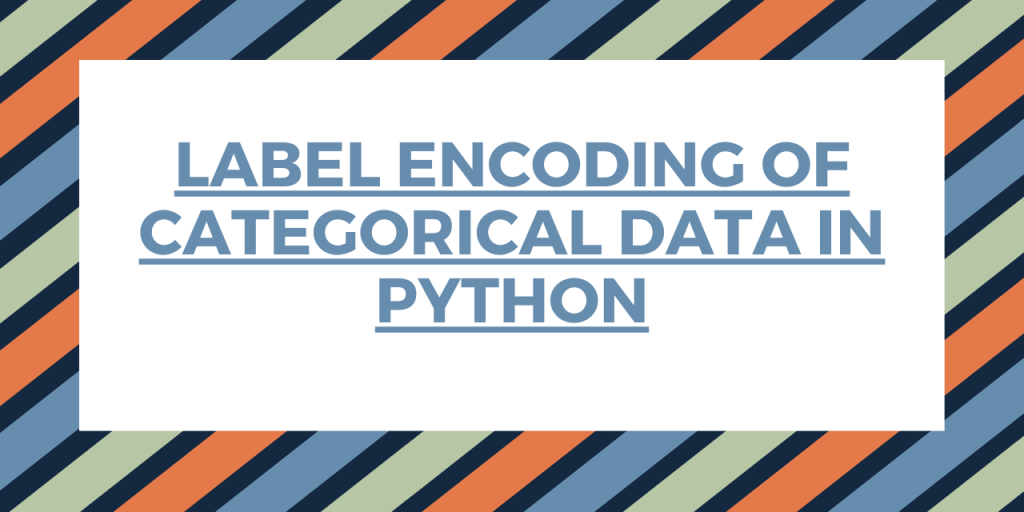# Label Encoding in Python – A Quick Guide!In our last article, we understood the working and implementation of One hot Encoding wherein Label Encoding is the initial step of the process.

Today, we’ll have a look at one of the most fundamental steps in the categorical encoding of data values.

So, without any further delay, let us begin!

## What is Label Encoding in Python?

Before diving deep into the concept of Label Encoding, let us understand the impact of the concept of ‘Label’ on the dataset.

A label is actually a number or a string that represents a particular set of entities. Labels helps the model in better understanding of the dataset and enables the model to learn more complex structures.

Recommended – How to standardize datasets for Machine learning?

Label Encoder performs the conversion of these labels of categorical data into a numeric format.

For example, if a dataset contains a variable ‘Gender’ with labels ‘Male’ and ‘Female’, then the label encoder would convert these labels into a number format and the resultant outcome would be [0,1].

Thus, by converting the labels into the integer format, the machine learning model can have a better understanding in terms of operating the dataset.

## Label Encoding – Syntax to know!

Python sklearn library provides us with a pre-defined function to carry out Label Encoding on the dataset.

Syntax:

```from sklearn import preprocessing
object = preprocessing.LabelEncoder()
```

Here, we create an object of the LabelEncoder class and then utilize the object for applying label encoding on the data.

### 1. Label Encoding with sklearn

Let’s get right into the process on label encoding. The first step to encoding a dataset is to have a dataset.

So, we’ll create a simple dataset here. Example: Creation of a dataset

```import pandas as pd
data = {"Gender":['M','F','F','M','F','F','F'], "NAME":['John','Camili','Rheana','Joseph','Amanti','Alexa','Siri']}
block = pd.DataFrame(data)
print("Original Data frame:\n")
print(block)
```

Here, we have created a dictionary ‘data’ and then transformed it into a DataFrame using `pandas.DataFrame() `function.

Output:

```Original Data frame:

Gender    NAME
0      M    John
1      F  Camili
2      F  Rheana
3      M  Joseph
4      F  Amanti
5      F   Alexa
6      F    Siri
```

From the above dataset, it is clear that the variable ‘Gender’ has labels as ‘M’ and ‘F’.

Further, now let us import the LabelEncoder class and applying it on the ‘Gender’ variable of the dataset.

```from sklearn import preprocessing
label = preprocessing.LabelEncoder()

block['Gender']= label.fit_transform(block['Gender'])
print(block['Gender'].unique())
```

We have used `fit_transform() method` to apply the functionality of the label encoder pointed by the object to the data variable.

Output:

```[1 0]
```

So, you see, the data has been transformed into integer labels of [0,1].

```print(block)
```

Output:

```Gender    NAME
0       1    John
1       0  Camili
2       0  Rheana
3       1  Joseph
4       0  Amanti
5       0   Alexa
6       0    Siri
```

### 2. Label Encoding using Category codes

Let us first check the data type of the variables of our dataset.

```block.dtypes
```

Data type:

```Gender    object
NAME      object
dtype: object
```

Now, transform and convert the datatype of the variable ‘Gender’ to category type.

```block['Gender'] = block['Gender'].astype('category')
```
```block.dtypes
```
```Gender    category
NAME        object
dtype: object
```

Now, let us transform the labels to integer types using `pandas.DataFrame.cat.codes` function.

```block['Gender'] = block['Gender'].cat.codes
```
```print(block)
```

As seen below, the variable ‘Gender’ has been encoded to integer values [0,1].

```Gender    NAME
0       1    John
1       0  Camili
2       0  Rheana
3       1  Joseph
4       0  Amanti
5       0   Alexa
6       0    Siri
```

## Conclusion

By this, we have come to the end of this topic. Feel free to comment below, in case you come across any question.

For a deeper understanding of the topic, try implementing the concept of Label Encoder on different dataset and variables. Do let us know your experience in the comment section ! 🙂

For more such posts related to Python, Stay tuned and till then, Happy Learning!! 🙂